# RS Aggarwal Solutions For Class 7 Maths Exercise 3A Chapter 3 Decimals

The pdf of RS Aggarwal Solutions for the Exercise 3A of Class 7 Maths Chapter 3, Decimals are given here in a simple but comprehensive way. Decimals solutions are extremely helpful for the students to clear all their doubts easily and understand the basics of this chapter more effectively. Students of Class 7 are suggested to solve RS Aggarwal Class 7 Solutions to strengthen the fundamentals and be able to solve questions that are usually asked in the examination.

RS Aggarwal Solutions For Class 7 Maths Chapter 3 Decimals Exercise 3A has problems which are based on comparing decimals, method of converting a decimal into a fraction and converting a fraction into a decimal. Students are suggested to try solving the questions from RS Aggarwal Solutions book of Class 7 and then refer to these solutions to know the best way of approaching different problems.

## Download the PDF of RS Aggarwal Solutions For Class 7 Maths Chapter 3 Decimals – Exercise 3A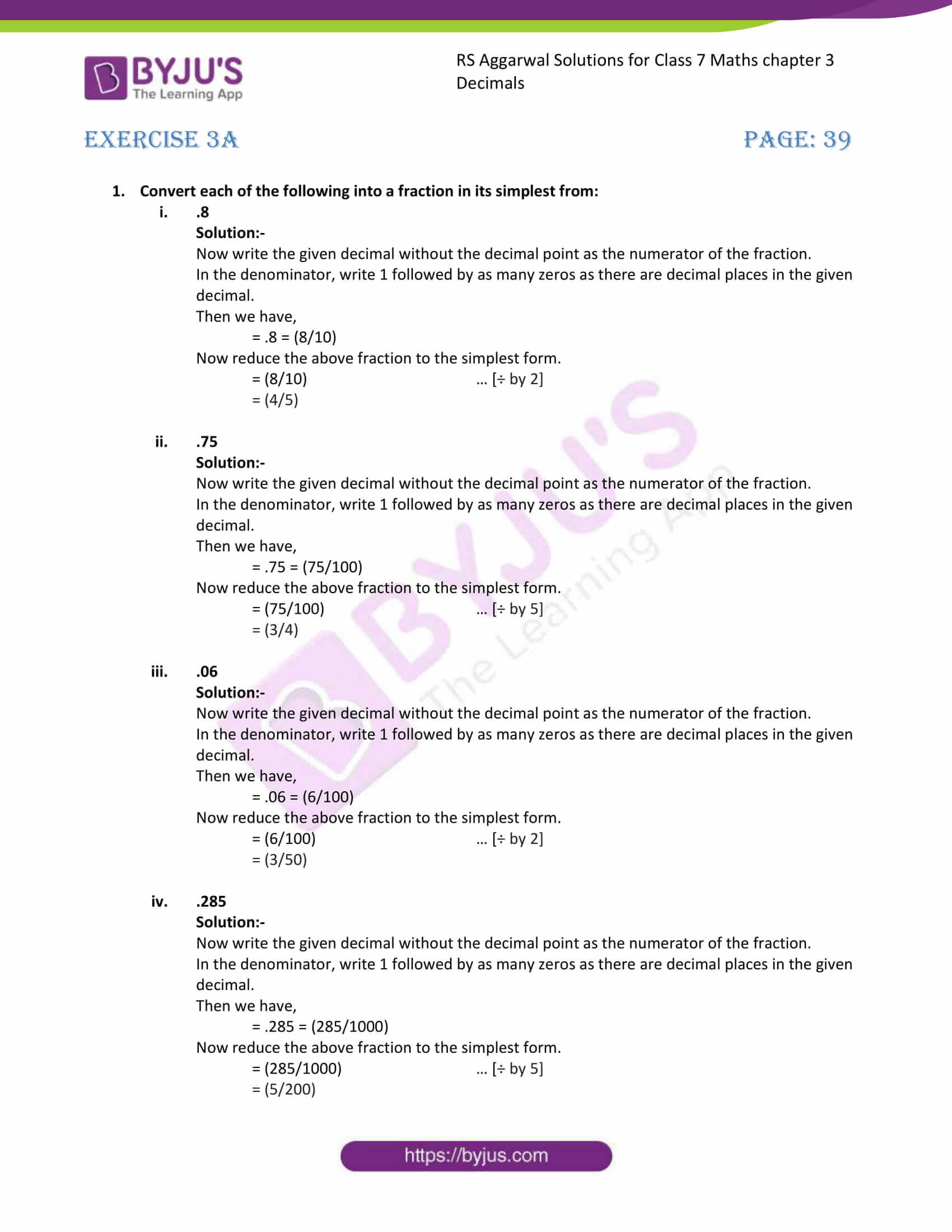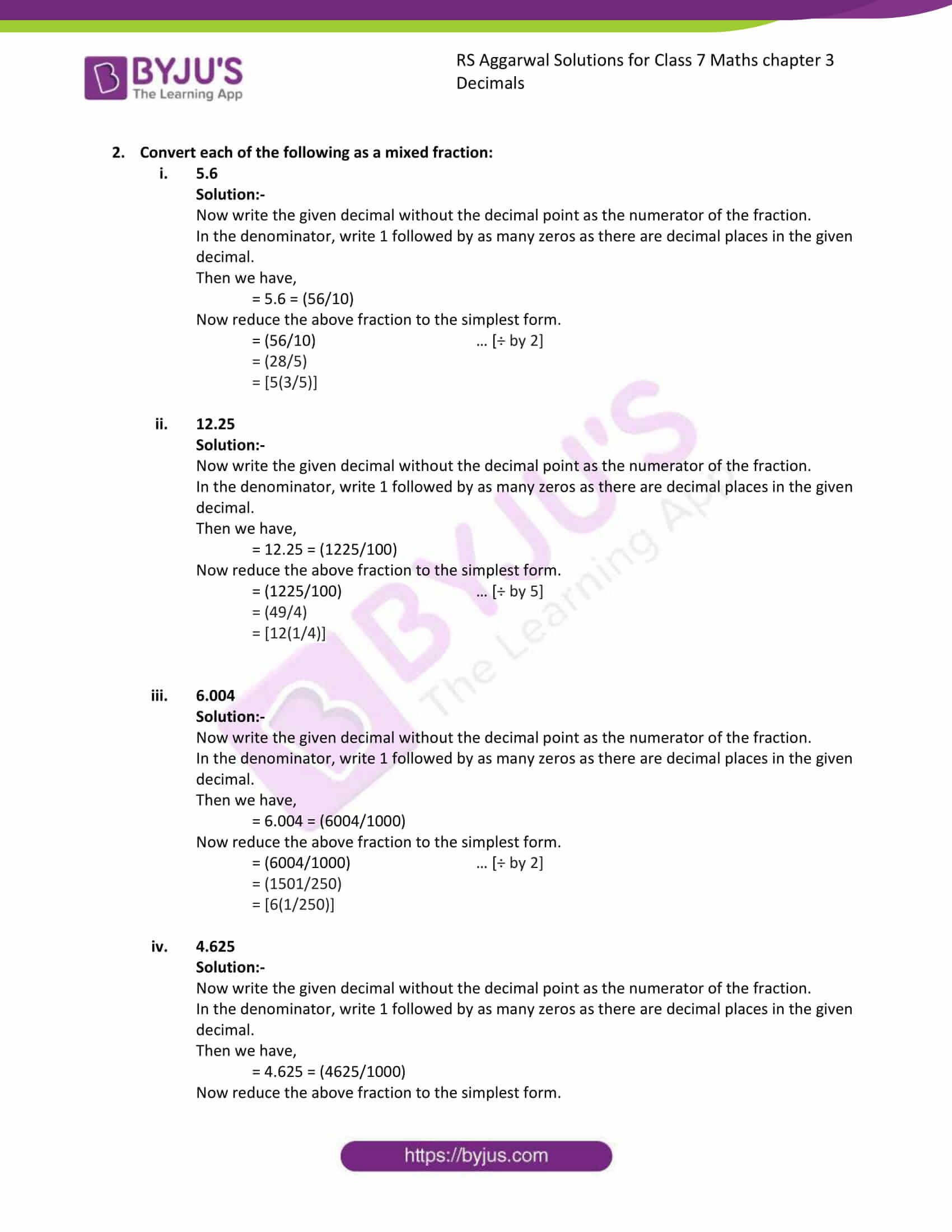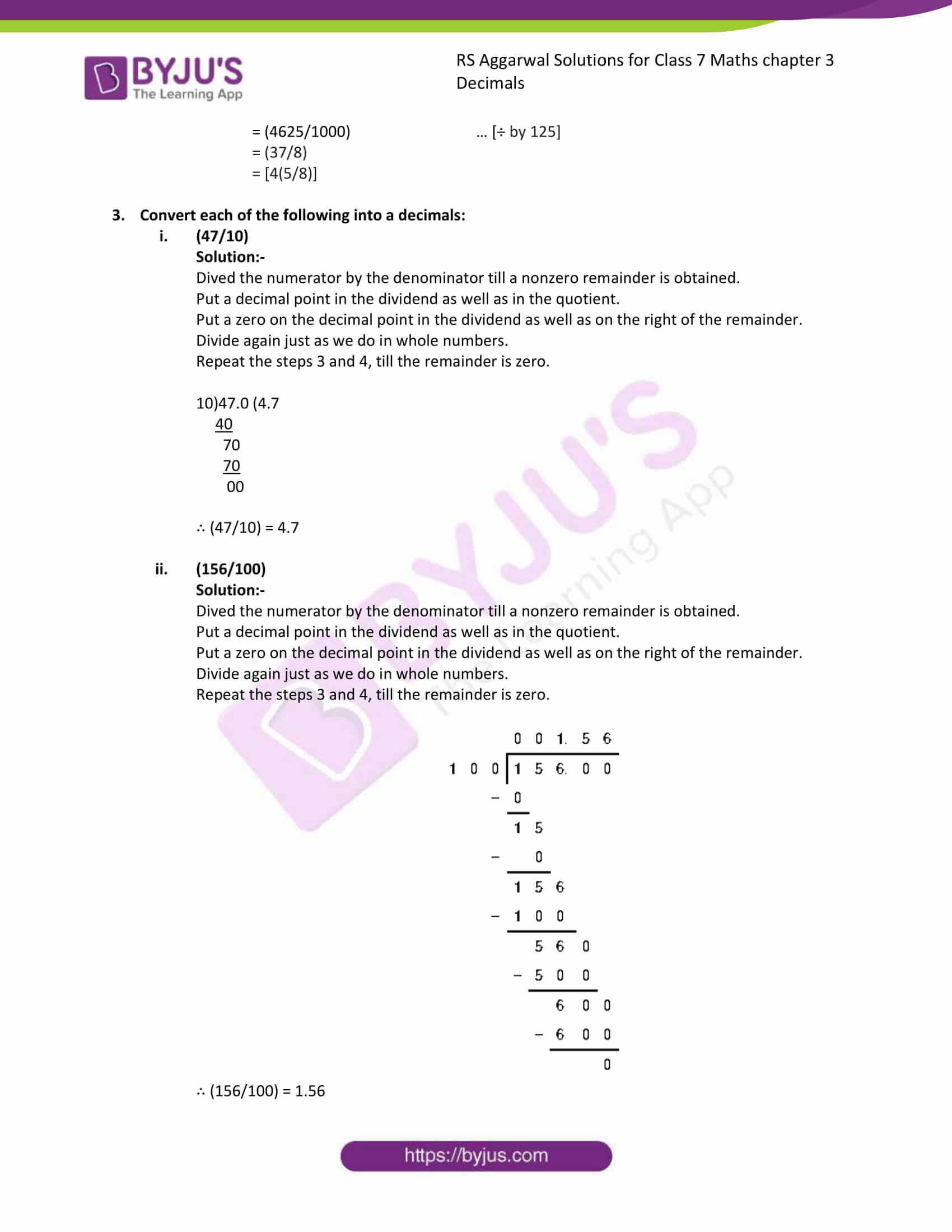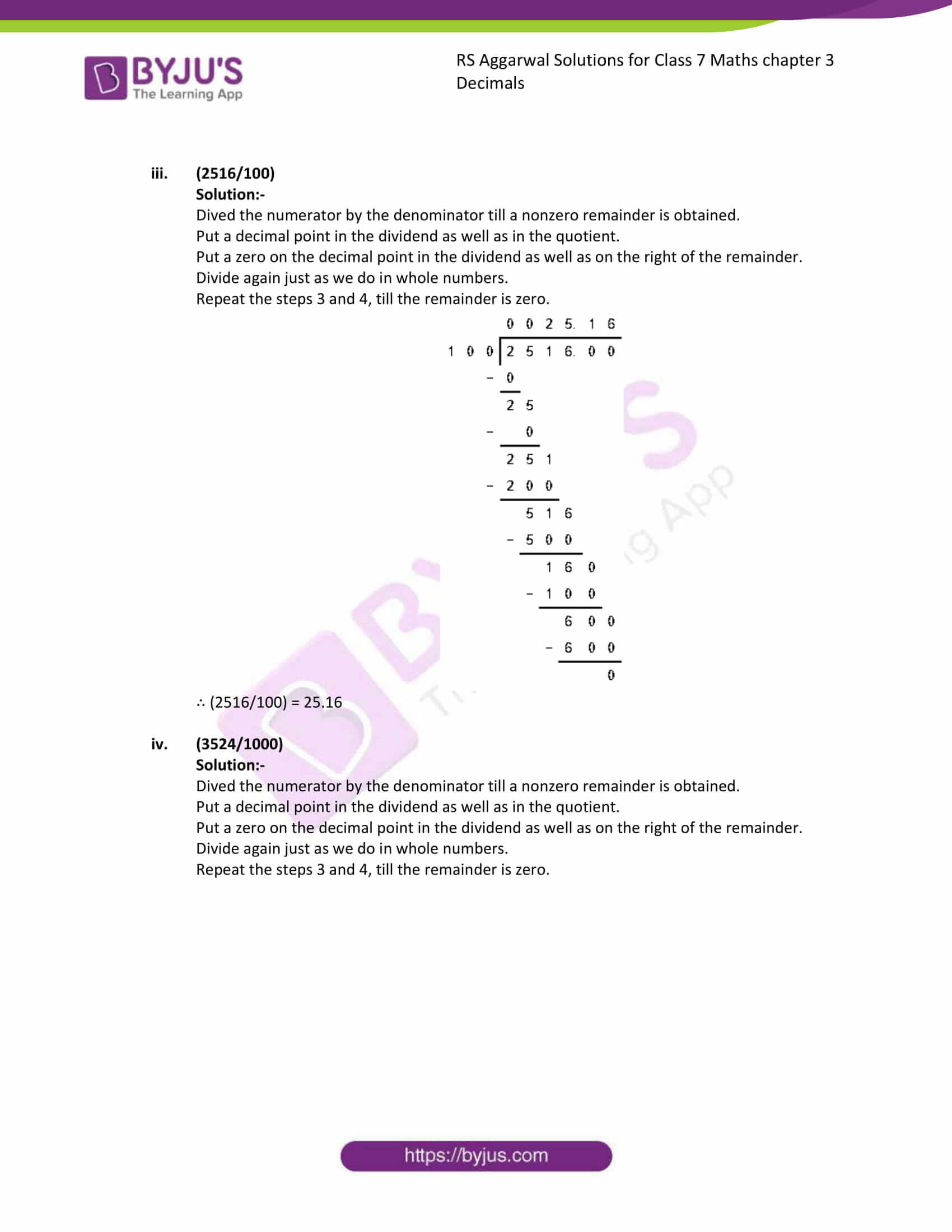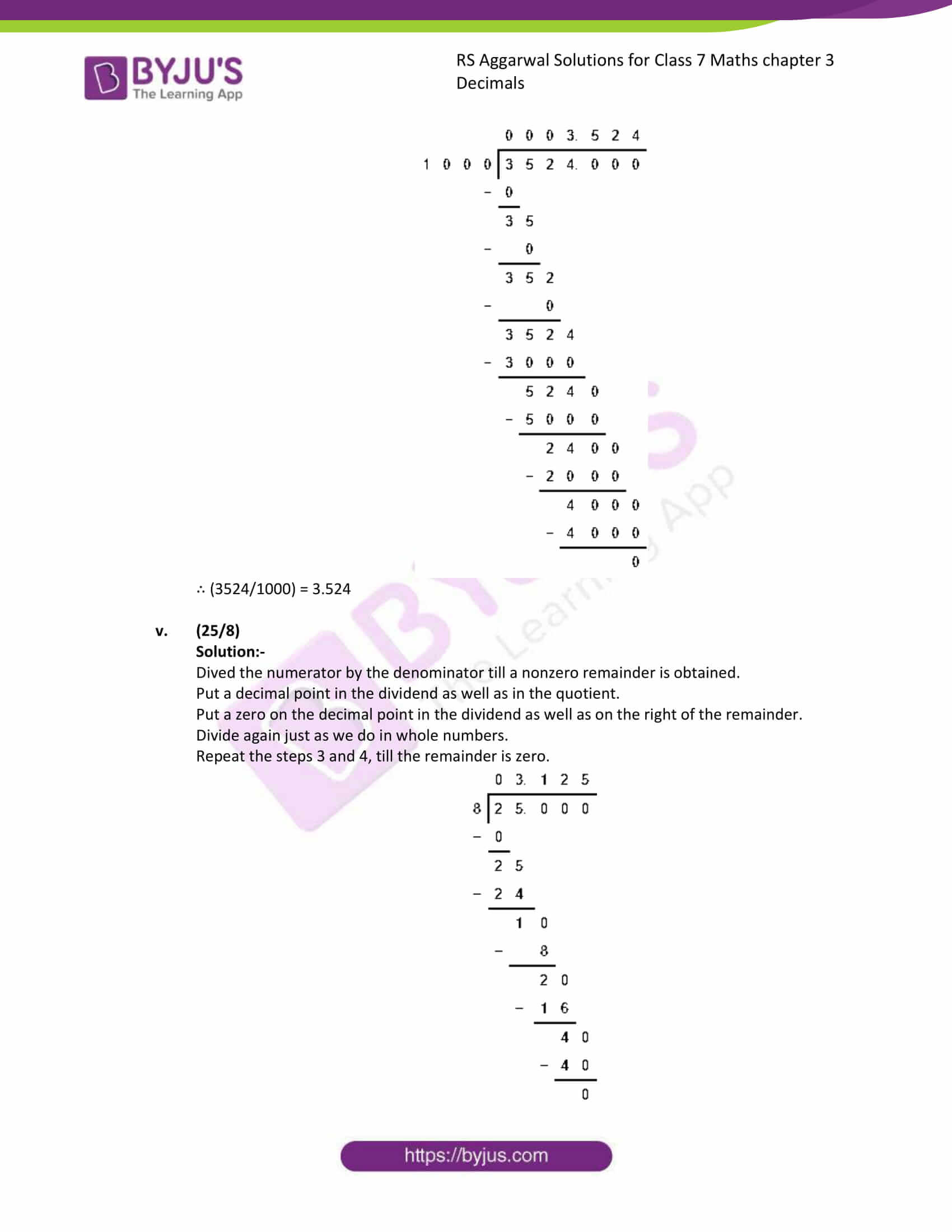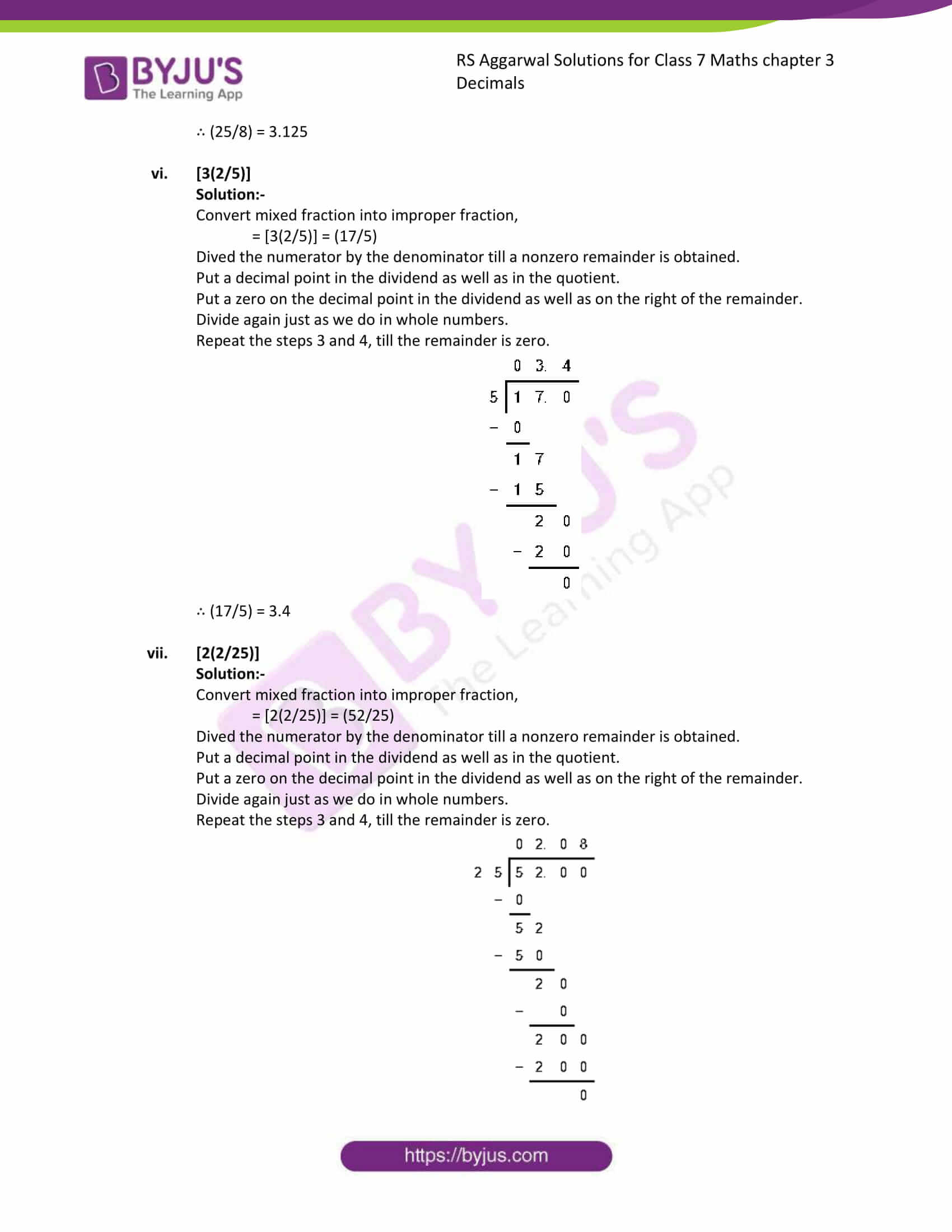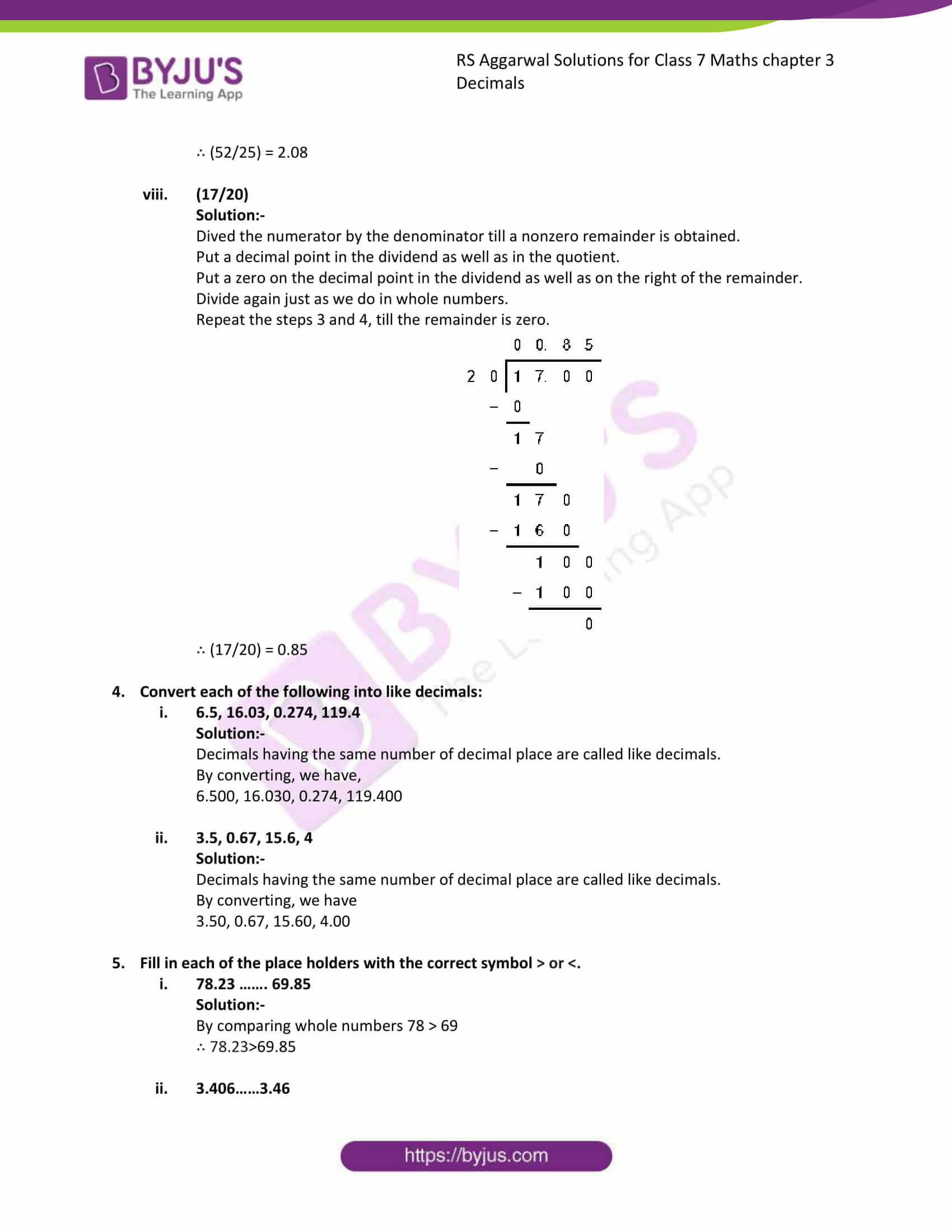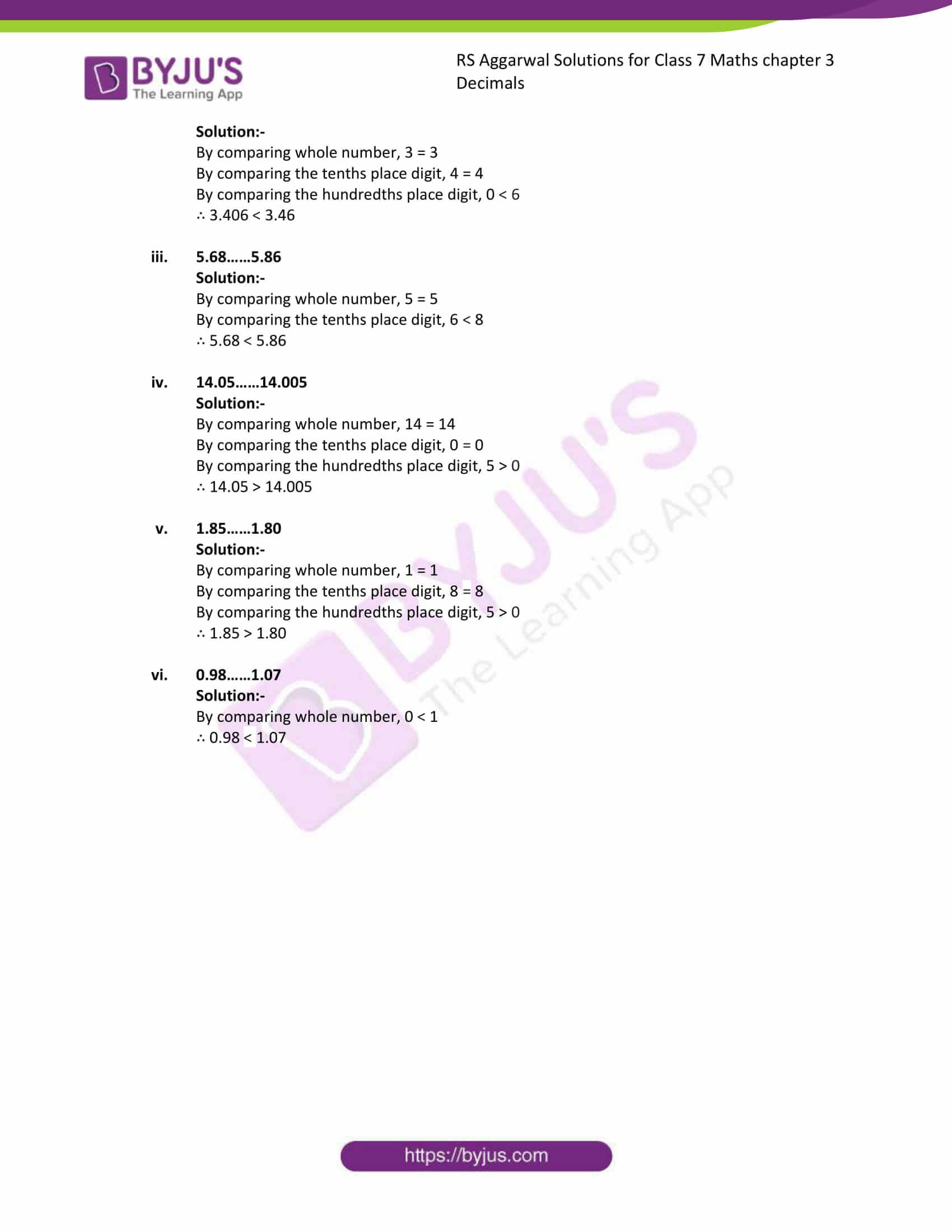### Access answers to Maths RS Aggarwal Solutions for Class 7 Chapter 3 – Decimals Exercise 3A

Convert each of the following into a fraction in its simplest from:

(i) .8

Solution:-

Now write the given decimal without the decimal point as the numerator of the fraction.

In the denominator, write 1 followed by as many zeros as there are decimal places in the given decimal.

Then we have,

= .8 = (8/10)

Now reduce the above fraction to the simplest form.

= (8/10) … [÷ by 2]

= (4/5)

(ii) .75

Solution:-

Now write the given decimal without the decimal point as the numerator of the fraction.

In the denominator, write 1 followed by as many zeros as there are decimal places in the given decimal.

Then we have,

= .75 = (75/100)

Now reduce the above fraction to the simplest form.

= (75/100) … [÷ by 5]

= (3/4)

(iii) .06

Solution:-

Now write the given decimal without the decimal point as the numerator of the fraction.

In the denominator, write 1 followed by as many zeros as there are decimal places in the given decimal.

Then we have,

= .06 = (6/100)

Now reduce the above fraction to the simplest form.

= (6/100) … [÷ by 2]

= (3/50)

(iv) .285

Solution:-

Now write the given decimal without the decimal point as the numerator of the fraction.

In the denominator, write 1 followed by as many zeros as there are decimal places in the given decimal.

Then we have,

= .285 = (285/1000)

Now reduce the above fraction to the simplest form.

= (285/1000) … [÷ by 5]

= (5/200)

2. Convert each of the following as a mixed fraction:

(i) 5.6

Solution:-

Now write the given decimal without the decimal point as the numerator of the fraction.

In the denominator, write 1 followed by as many zeros as there are decimal places in the given decimal.

Then we have,

= 5.6 = (56/10)

Now reduce the above fraction to the simplest form.

= (56/10) … [÷ by 2]

= (28/5)

= [5(3/5)]

(ii) 12.25

Solution:-

Now write the given decimal without the decimal point as the numerator of the fraction.

In the denominator, write 1 followed by as many zeros as there are decimal places in the given decimal.

Then we have,

= 12.25 = (1225/100)

Now reduce the above fraction to the simplest form.

= (1225/100) … [÷ by 5]

= (49/4)

= [12(1/4)]

(iii) 6.004

Solution:-

Now write the given decimal without the decimal point as the numerator of the fraction.

In the denominator, write 1 followed by as many zeros as there are decimal places in the given decimal.

Then we have,

= 6.004 = (6004/1000)

Now reduce the above fraction to the simplest form.

= (6004/1000) … [÷ by 2]

= (1501/250)

= [6(1/250)]

(iv) 4.625

Solution:-

Now write the given decimal without the decimal point as the numerator of the fraction.

In the denominator, write 1 followed by as many zeros as there are decimal places in the given decimal.

Then we have,

= 4.625 = (4625/1000)

Now reduce the above fraction to the simplest form.

= (4625/1000) … [÷ by 125]

= (37/8)

= [4(5/8)]

3. Convert each of the following into a decimals:

(i) (47/10)

Solution:-

Dived the numerator by the denominator till a nonzero remainder is obtained.

Put a decimal point in the dividend as well as in the quotient.

Put a zero on the decimal point in the dividend as well as on the right of the remainder.

Divide again just as we do in whole numbers.

Repeat the steps 3 and 4, till the remainder is zero.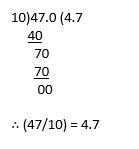(ii) (156/100)

Solution:-

Dived the numerator by the denominator till a nonzero remainder is obtained.

Put a decimal point in the dividend as well as in the quotient.

Put a zero on the decimal point in the dividend as well as on the right of the remainder.

Divide again just as we do in whole numbers.

Repeat the steps 3 and 4, till the remainder is zero.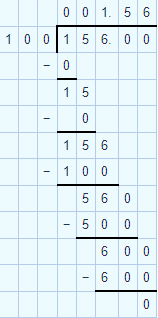∴ (156/100) = 1.56

(iii) (2516/100)

Solution:-

Dived the numerator by the denominator till a nonzero remainder is obtained.

Put a decimal point in the dividend as well as in the quotient.

Put a zero on the decimal point in the dividend as well as on the right of the remainder.

Divide again just as we do in whole numbers.

Repeat the steps 3 and 4, till the remainder is zero.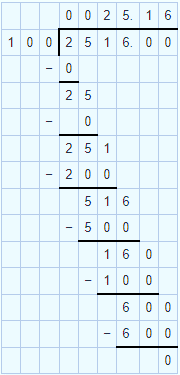∴ (2516/100) = 25.16

(iv) (3524/1000)

Solution:-

Dived the numerator by the denominator till a nonzero remainder is obtained.

Put a decimal point in the dividend as well as in the quotient.

Put a zero on the decimal point in the dividend as well as on the right of the remainder.

Divide again just as we do in whole numbers.

Repeat the steps 3 and 4, till the remainder is zero.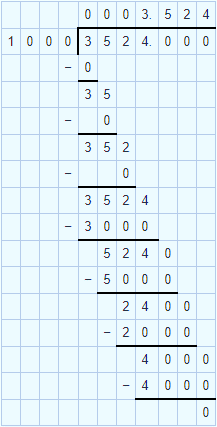∴ (3524/1000) = 3.524

(v) (25/8)

Solution:-

Dived the numerator by the denominator till a nonzero remainder is obtained.

Put a decimal point in the dividend as well as in the quotient.

Put a zero on the decimal point in the dividend as well as on the right of the remainder.

Divide again just as we do in whole numbers.

Repeat the steps 3 and 4, till the remainder is zero.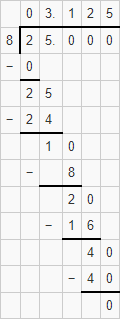∴ (25/8) = 3.125

(vi) [3(2/5)]

Solution:-

Convert mixed fraction into improper fraction,

= [3(2/5)] = (17/5)

Dived the numerator by the denominator till a nonzero remainder is obtained.

Put a decimal point in the dividend as well as in the quotient.

Put a zero on the decimal point in the dividend as well as on the right of the remainder.

Divide again just as we do in whole numbers.

Repeat the steps 3 and 4, till the remainder is zero.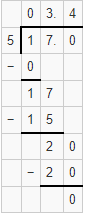∴ (17/5) = 3.4

(vii) [2(2/25)]

Solution:-

Convert mixed fraction into improper fraction,

= [2(2/25)] = (52/25)

Dived the numerator by the denominator till a nonzero remainder is obtained.

Put a decimal point in the dividend as well as in the quotient.

Put a zero on the decimal point in the dividend as well as on the right of the remainder.

Divide again just as we do in whole numbers.

Repeat the steps 3 and 4, till the remainder is zero.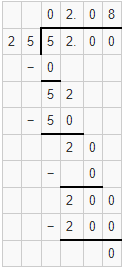∴ (52/25) = 2.08

(viii) (17/20)

Solution:-

Dived the numerator by the denominator till a nonzero remainder is obtained.

Put a decimal point in the dividend as well as in the quotient.

Put a zero on the decimal point in the dividend as well as on the right of the remainder.

Divide again just as we do in whole numbers.

Repeat the steps 3 and 4, till the remainder is zero.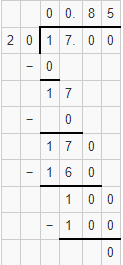∴ (17/20) = 0.85

4. Convert each of the following into like decimals:

(i) 6.5, 16.03, 0.274, 119.4

Solution:-

Decimals having the same number of decimal place are called like decimals.

By converting, we have,

6.500, 16.030, 0.274, 119.400

(ii) 3.5, 0.67, 15.6, 4

Solution:-

Decimals having the same number of decimal place are called like decimals.

By converting, we have

3.50, 0.67, 15.60, 4.00

5. Fill in each of the place holders with the correct symbol > or <.

(i) 78.23 ……. 69.85

Solution:-

By comparing whole numbers 78 > 69

∴ 78.23>69.85

(ii) 3.406……3.46

Solution:-

By comparing whole number, 3 = 3

By comparing the tenths place digit, 4 = 4

By comparing the hundredths place digit, 0 < 6

∴ 3.406 < 3.46

(iii) 5.68……5.86

Solution:-

By comparing whole number, 5 = 5

By comparing the tenths place digit, 6 < 8

∴ 5.68 < 5.86

(iv) 14.05……14.005

Solution:-

By comparing whole number, 14 = 14

By comparing the tenths place digit, 0 = 0

By comparing the hundredths place digit, 5 > 0

∴ 14.05 > 14.005

(v) 1.85……1.80

Solution:-

By comparing whole number, 1 = 1

By comparing the tenths place digit, 8 = 8

By comparing the hundredths place digit, 5 > 0

∴ 1.85 > 1.80

(vi) 0.98……1.07

Solution:-

By comparing whole number, 0 < 1

∴ 0.98 < 1.07

### Access other exercises of RS Aggarwal Solutions For Class 7 Chapter 3 – Decimals

Exercise 3E Solutions 21 Questions